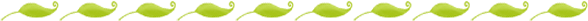Number Sense and the Common Core: Part-Whole Relationships

In Part 1 of this series I discussed how important number sense is to a child's development along with early number sense skills and how they relate to the Common Core. In Part 2 I discussed the importance of hierarchal inclusion, magnitude, and subitizing. The whole of Common Core math (except for geometry and measurement) fall under the umbrella of number sense, and today we will discuss the aspect of number sense that should be the major focus of any kindergarten math program.Part/Whole Relationships

"Count out a set of eight counters... [a]ny child who has learned how to count meaningfully can count out eight objects as you just did. What is significant about the experience is what it did not cause you to think about. Nothing in counting a set of eight objects will cause a child to focus on the fact that it could be made of two parts. For example, separate the counters you just set out into two piles and reflect on the combination. It might be 2 and 6 or 7 and 1 or 4 and 4. Make a change in your two piles of counters and say the new combination to yourself. Focusing on a quantity in terms of its parts has important implications for developing number sense. The ability to think about a number in terms of parts is a major milestone in the development of number" (Walle and Lovin 2006).

All of the following Common Core standards involve an understanding of part/whole relationships. Notice that the word equation is mentioned in only three of these standards, and in those standards it is only one option that students can use to represent addition and subtraction. In actuality, using an equation may not be developmentally appropriate for most kindergartners. What they should be doing in order to meet the standard, is show addition and subtraction in terms of the part/whole relationships of numbers.

For example, if you ask a child to show combinations of 10 with colored plates, as shown in the previous video, and he/she can tell you all of the different combinations that make ten, they have just solved a problem involving addition to 10 or subtraction from 10 using a drawing. This meets Core Standard K.OA.A.3 without solving any abstract equations.

"To really understand addition and subtraction, we must understand how they are connected... By modeling addition and subtraction situations and then generalizing across these situations, children are able to understand and represent the operations of addition and subtraction... Children who commit the facts to memory easily are able to do so because they have constructed relationships among them and between addition and subtraction in general, and they use these relationships as shortcuts. When relationships are the focus, there are far fewer facts to remember, and big ideas like compensation, hierarchical inclusion, and part/whole relationships come into play. Also, if a child forgets an answer, she has a quick way to come up with it" (Fosnot and Dolk 2001)

CCSS.MATH.CONTENT.K.OA.A.1 Represent addition and subtraction with objects, fingers, mental images, drawings, sounds (e.g., claps), acting out situations, verbal explanations, expressions, or equations.

CCSS.MATH.CONTENT.K.OA.A.2 Solve addition and subtraction word problems, and add and subtract within 10, e.g., by using objects or drawings to represent the problem.

CCSS.MATH.CONTENT.K.OA.A.3 Decompose numbers less than or equal to 10 into pairs in more than one way, e.g., by using objects or drawings, and record each decomposition by a drawing or equation (e.g., 5 = 2 + 3 and 5 = 4 + 1).

CCSS.MATH.CONTENT.K.OA.A.4 For any number from 1 to 9, find the number that makes 10 when added to the given number, e.g., by using objects or drawings, and record the answer with a drawing or equation.

CCSS.MATH.CONTENT.K.OA.A.5 Fluently add and subtract within 5.

It would be difficult to overstate how important part/whole relationships are to a kindergartener's mathematical development. I highly recommend looking into the books that I have used as sources in these discussions if you would like more information as well as some great lessons on teaching part/whole relationships. Our Interactive Math Worksheets for March  and Interactive Math Worksheets for January also include some activities designed to develop this skill. Tomorrow we will discuss some of the number sense skills that are on the horizon for kindergartners.